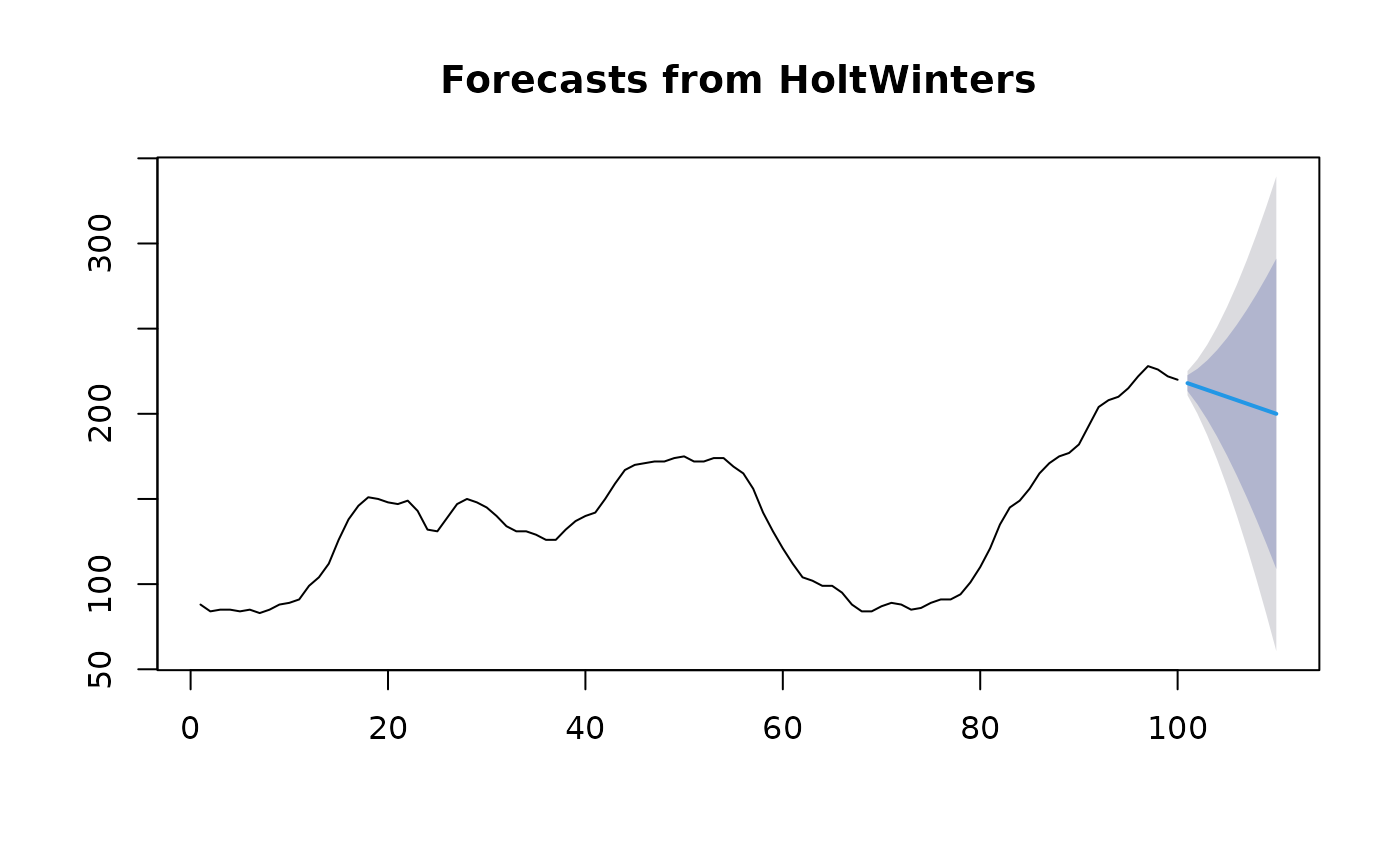Returns forecasts and other information for univariate Holt-Winters time series models.

# S3 method for HoltWinters
forecast(
object,
h = ifelse(frequency(object$x) > 1, 2 * frequency(object$x), 10),
level = c(80, 95),
fan = FALSE,
lambda = NULL,
...
)

## Arguments

object An object of class "HoltWinters". Usually the result of a call to HoltWinters. Number of periods for forecasting Confidence level for prediction intervals. If TRUE, level is set to seq(51,99,by=3). This is suitable for fan plots. Box-Cox transformation parameter. If lambda="auto", then a transformation is automatically selected using BoxCox.lambda. The transformation is ignored if NULL. Otherwise, data transformed before model is estimated. Use adjusted back-transformed mean for Box-Cox transformations. If transformed data is used to produce forecasts and fitted values, a regular back transformation will result in median forecasts. If biasadj is TRUE, an adjustment will be made to produce mean forecasts and fitted values. Other arguments.

## Value

An object of class "forecast".

The function summary is used to obtain and print a summary of the results, while the function plot produces a plot of the forecasts and prediction intervals.

The generic accessor functions fitted.values and residuals extract useful features of the value returned by forecast.HoltWinters.

An object of class "forecast" is a list containing at least the following elements:

model

A list containing information about the fitted model

method

The name of the forecasting method as a character string

mean

Point forecasts as a time series

lower

Lower limits for prediction intervals

upper

Upper limits for prediction intervals

level

The confidence values associated with the prediction intervals

x

The original time series (either object itself or the time series used to create the model stored as object).

residuals

Residuals from the fitted model.

fitted

Fitted values (one-step forecasts)

## Details

This function calls predict.HoltWinters and constructs an object of class "forecast" from the results.

It is included for completeness, but the ets is recommended for use instead of HoltWinters.

predict.HoltWinters, HoltWinters.
fit <- HoltWinters(WWWusage,gamma=FALSE)